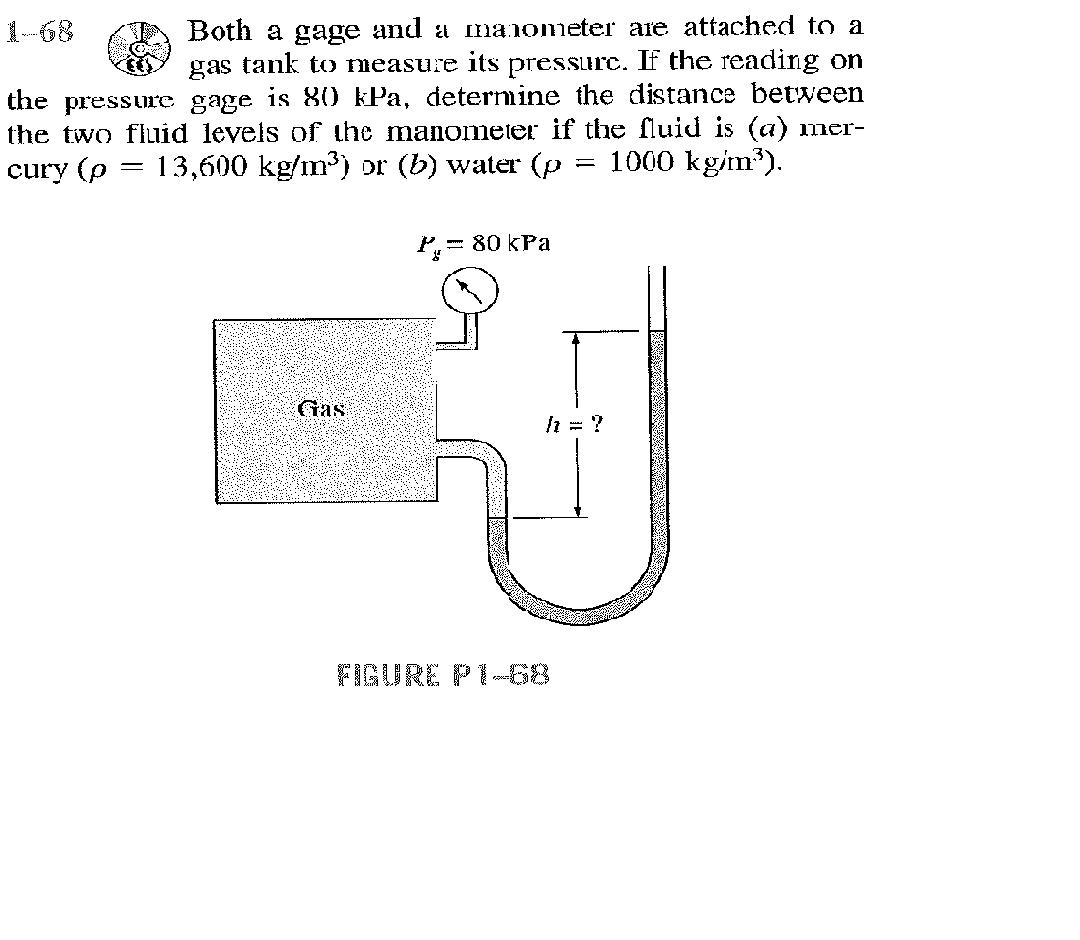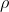# Both a gage and a manometer are attached to a gas tank to measure its pressure. If the reading on the pressure gage is 80 kPa, determine the distance between the two fluid levels of the manometer if the fluid is (a) mercury (p = 13,600 kg/m3) or (b) water (p = 1000 kg/m3)

Question-AnswerCategory: Fluid MechanicsBoth a gage and a manometer are attached to a gas tank to measure its pressure. If the reading on the pressure gage is 80 kPa, determine the distance between the two fluid levels of the manometer if the fluid is (a) mercury (p = 13,600 kg/m3) or (b) water (p = 1000 kg/m3)

Both a gage and a manometer are attached to a gas tank to measure its pressure. If the reading on the pressure gage is 80 kPa, determine the distance between the two fluid levels of the manometer if the fluid is (a) mercury (p = 13,600 kg/m3) or (b) water (p = 1000 kg/m3)Pg=80*10^3 N/m^2

we know  Pg =ρgH

ρ= Density of the measuring liquid

(a) For Mercury
Pg= ρgH
80*10^3 =13600*9.81*H
H=0.599m

(b) For  water

Pg= ρgH
80*10^3 =1000*9.81*H
H=8.15m

a)  pressure =gH
80000 = 13600×9.8xH
H = 0.6 m

b)  80000= 1000×9.8xH

H = 8.16 m